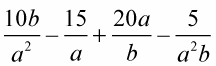##### Vegetable Gardening For Dummies, 2nd EditionDistributing with negative exponents means that you'll have fractional answers. A base that has a negative exponent can be changed to a fraction. The base and the exponent become the denominator, but the exponent loses its negative sign in the process. You then cap it all off with a 1 in the numerator.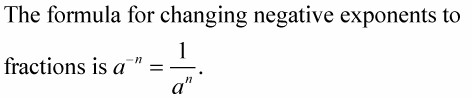The following steps show how a negative exponent can lead to a fraction.

1. Distribute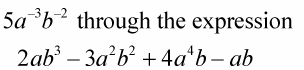Here's what you'll get.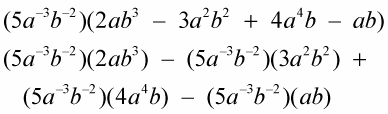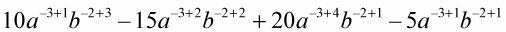3. The factor of b with the 0 exponent becomes 1.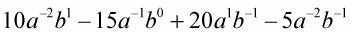4. Change terms with negative exponents to fractions.